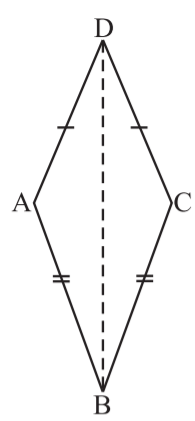Question

Updated on:30/05/2023

# In the given figure, AD=CDandAB=CB. (i) state the three pairs of equal in ΔABDandΔCBD. (iii) Is ΔABD[≡~?]ΔCBD ? Why or why not ? (iii) Does BD bisect ? ABC ? Given reasonsText Solution

Solution
(i) In ABD and CBD, the three pairs of equal parts are as given below:
AB=CB (Given)
AD=CD (Given) and BD=BD (Common in both)
(ii) From (i) above, ABDCBD (By SSS congruence rule)
(iii)ABD=CBD (Corresponding parts of congruent triangles) So, BD bisects ABC.

Transcript

in example 3 it is given that ad is equal to CD is equal to CBE state first state three pairs of equal parts in triangle abc and triangle CBD equal parts kaun kaun se ho gaye to CD ab = to see be given aur Bidi Quest to BD common Shayad 3 equal parts Ho Gaye S parties is triangle ABC congruent to triangle CBD triangle are congruent to triangle CBD Kaise dekhte hain to abe
cbk khol ke Khol Hai Bidi Bidi common hai and is equals to Shirdi so they are congruent by excess congruency yes they are congruent kya bhai XXX congressi last part kya hai does BD bisects triangle ABC and bisect triangle ABC Kyunki donon congruent to triangle ABC is congruent to triangle c b d
Sewing ke half be congruent Honge triangle ABC is copper Marne the point to ab is congruent to triangle CB Hoga half hour with same by excess so congruent iska matlab kya hoga equals to see this implies equals to see you Hoga Kabhi congruent hai donon so iska matlab Agar apan triangle Dekhe now now in triangle abc Agar ABC triangle lekin yah wala to aap bhi Basa kar raha hai Kyunki donon cal Hi Ho
is bisecting side AC because of this so we can say that BD bisect angle abc this implies it is also bisecting Triangle video